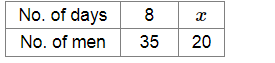# Mark (✓) against the correct answer:

Question:

Mark (✓) against the correct answer:

35 men can reap a field in 8 days. In how many days can 20 men reap it?

(a) 14 days

(b) 28 days

(c) $87 \frac{1}{2}$ days

(d) none of these

Solution:

(a) 14 days

Let 20 men take days to reap the field.Clearly, less number of men will take more days.

So, it is a case of inverse proportion.

Now, $8 \times 35=x \times 20$

$\Rightarrow x=\frac{8 \times 35}{20}$

$\Rightarrow x=14$

∴ 20 men can reap the field in 14 days.